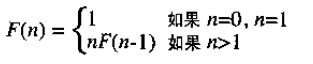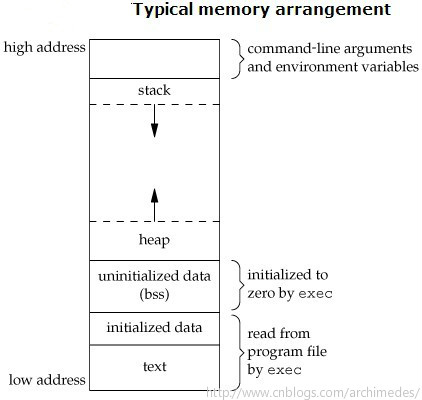# 在计算机科学领域中，递归式通过递归函数来实现的。程序调用自身的编程技巧称为递归（ recursion）。 一个过程或函数在其定义或说明中有直接或间接调用自身的一种方法，它通常把一个大型复杂的问题层层转化为一个与原问题相似的规模较小的问题来求解， 递归策略只需少量的程序就可描述出解题过程所需要的多次重复计算，大大地减少了程序的代码量。递归的能力在于用有限的语句来定义对象的无限集合。 一般来说，递归需要有：边界条件、递归前进段和递归返回段。 当边界条件不满足时，递归前进；当边界条件满足时，递归返回。 注意： (1) 递归就是在过程或函数里调用自身； (2) 在使用递归策略时，必须有一个明确的递归结束条件，称为递归出口。 本文地址：http://www.cnblogs.com/archimedes/p/rescuvie-tailrescuvie.html，转载请注明源地址。

## 基本递归F(4)=4×F(3)　　　　　　　　　　　　递归阶段

F(3)=3×F(2)

F(2)=2×F(1)

F(1)=1　　终止条件

F(2)=(2)×(1)　　　 回归阶段

F(3)=(3)×(2)

F(4)=(4)×(6)

24　　　　　　　　　　　　　　　　  递归完成

```

int fact(int n) {    if(n < 0)        return 0;    else if (n == 0 || n == 1)        return 1;    else

return n * fact(n - 1);

}```

BSS段:（bss segment）通常是指用来存放程序中未初始化的全局变量的一块内存区域。BSS是英文Block Started by Symbol的简称。BSS段属于静态内存分配。```

#include <stdio.h>int g1=0, g2=0, g3=0;int max(int i)

{    int m1 = 0, m2, m3 = 0, *p_max;    static n1_max = 0, n2_max, n3_max = 0;

p_max = (int*)malloc(10);
printf("打印max程序地址\n");

参数地址\n");     printf("in max: 0x%08x\

printf("in max: 0x%08x\n\n",max);
printf("打印max传

printf("打印max函数中静态变量地址\n");
printf("0x%08x\n",&n1_max); //打印各本地变量的内存地址

地址\n");     printf("0x%08x\n",&

printf("0x%08x\n",&n2_max);
printf("0x%08x\n\n",&n3_max);
printf("打印max函数中局部变

printf("0x%08x\n",&m2);
printf("0x%08x\n\n",&m3);
printf("打印max函数中malloc分配地址\n");

v) {    static int s1=0, s2, s3=0;    int v1=0

printf("0x%08x\n\n",p_max); //打印各本地变量的内存地址
if(i) return 1;    else return 0;
}int main(int argc, char **ar
g, v2, v3=0;    int *p;
p = (int*)malloc(10);
printf("打印各全局变量(已初始化)的内存地址\n");
printf("0x%08x\n",&g1); //打印各全局变量的内存地址
printf("0x%08x\n",&g2);

);     printf("argv: 0x%08x\n

printf("0x%08x\n\n",&g3);
printf("======================\n");
printf("打印程序初始程序main地址\n");
printf("main: 0x%08x\n\n", main);
printf("打印主参地址\n
"\n",argv);
printf("打印各静态变量的内存地址\n");
printf("0x%08x\n",&s1); //打印各静态变量的内存地址
printf("0x%08x\n",&s2);
printf("0x%08x\n\n",&s3);
printf("打印各局部变量的内存地址\n");

printf("======================\n");     ma

printf("0x%08x\n",&v1); //打印各本地变量的内存地址
printf("0x%08x\n",&v2);
printf("0x%08x\n\n",&v3);
printf("打印malloc分配的堆地址\n");
printf("malloc: 0x%08x\n\n",p);

x(v1);
printf("======================\n");
printf("打印子函数起始地址\n");
printf("max: 0x%08x\n\n",max);    return 0;

}```

## 尾递归

### 实例

```

int facttail(int n, int a)

{    if (n < 0)        return 0;    else if (n == 0)        return 1;    else if (n == 1)        return a;    else

return facttail(n - 1, n * a);

}```